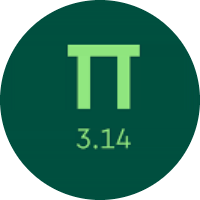# Learning the Decimal Numbers and their Operations

In this quiz, you will briefly learn about the concept of Decimal. you will find problems of basic mathematical operations with decimal numbers. This quiz will surely help you in practicing decimal numbers.

Start Quiz

Put these numbers in order from least to greatest.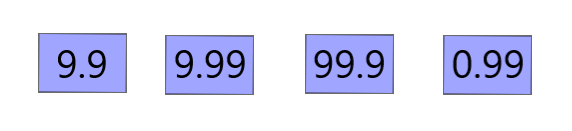0.99<9.9<9.99<99.9

9.9<0.99<9.99<99.9

0.99<9.99<9.9<99.9

Which sign makes the statement true?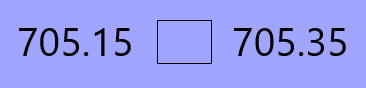<

>

=

Evaluate.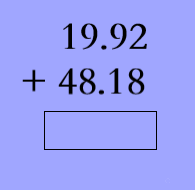68.30

68.10

58.10

Subtract.48.2

68.2

48.4

Find the missing decimal number.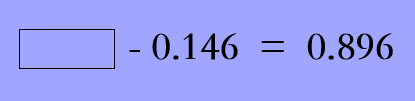1.420

2.042

1.042

Select the sign that makes the statement true.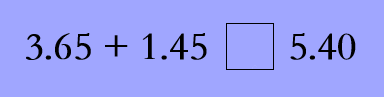=

>

<

What is 61.184 rounded to the nearest whole number?

61

62

60

Which fraction is about to equal 0.333?

10/3

2/3

1/3

Jenna runs 9.34 kilometers every morning. One day, she runs 2.08 kilometers and then stops to take a break. How much further does Jenna have left to run?

5.66 kilometers

7.26 kilometers

8.62 kilometers

Quiz/Test Summary
Title: Learning the Decimal Numbers and their Operations
Questions: 9
Contributed by: